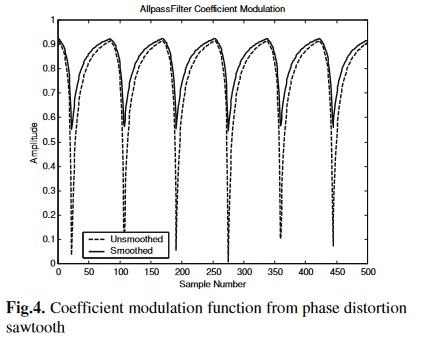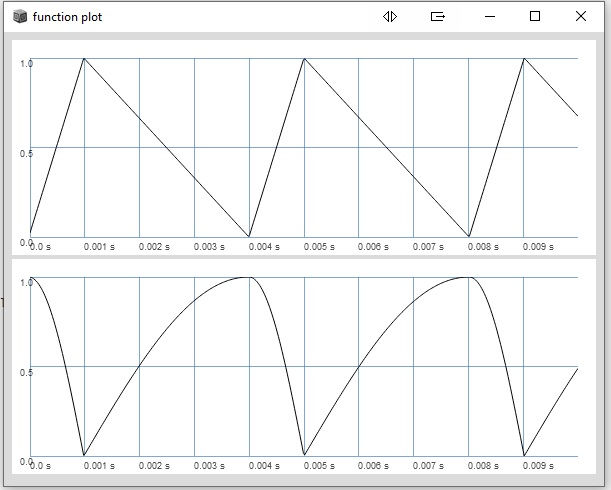# Phase Distortion with Fb1

hey, is there someone who could help me adjusting this Fb1 Allpass Example so it could be used for phase distortion by modulation the coefficient of an first-order allpass filter as presented in chapter 4 “phase distortion with allpass coefficient modulation” of this paper https://www.researchgate.net/publication/220733360_Spectrally_rich_phase_distortion_sound_synthesis_using_an_allpass_filter

The function for the filter is already fine, but im not sure how to implement the coefficient modulation function in fig. 4.``````(
{
var d = 10;
var g = MouseX.kr(0.01, 0.99).poll;
var sig = Saw.ar(50, 0.1);
var allp = Fb1(
{ |in, out| (g.neg * in) + in[d] + (g * out[d]) },
sig,
inDepth: d + 1, outDepth: d + 1,
blockSize: s.options.blockSize
);
sig + allp ! 2
}.play
)
``````

i think the variable slope ramp is already getting somewhere

``````//variable-slope ramp
(
{
var width = 0.25;
var freq = 400;
var sine;
var phasor = LFSaw.ar(freq/2, 1).linlin(-1,1, width.neg, 1-width);
phasor = phasor.bilin(0, width.neg, 1-width, 0, -0.5, 1);
sine = (Select.ar(phasor>0, [0.5+phasor, phasor])*2pi).sin.fold(0,1);

[phasor, sine]
}.plot
)
``````

this looks already “sawish”. any further ideas?

``````// first-order Allpass Coefficient Modulation for Phase Distortion Synthesis

(
{
var d = 2;
var width = 0.20;
var freq = 400;
var coef, allp;
var sig = SinOsc.ar(freq);
var phasor = LFSaw.ar(freq/2, 1).linlin(-1,1, width.neg, 1-width);
phasor = phasor.bilin(0, width.neg, 1-width, 0, -0.5, 1);
coef = (Select.ar(phasor>0, [0.5+phasor, phasor])*2pi).sin.fold(0,1);

allp = Fb1(
{ |in, out| (coef.neg * in) + in[d] + (coef * out[d]) },
sig,
inDepth: d + 1, outDepth: d + 1,
blockSize: s.options.blockSize
);

[phasor, coef, allp]
}.plot
)
``````

Hey,

the problem here is this: you’re taking over `coef` directly in the Fb1 function, hence it’s only kr, due to the way the Fb1 func is used. If you want it at ar you’d have to pass it with the `in` arg. That’s an important piece of information that maybe goes under a bit in the lengthy help file (although it’s mentioned and used in several examples).

I did not analyze your modulator but the result now looks smooth:

``````

(
s.options.blockSize = 8;
s.quit.reboot;
)

(
{
var d = 2;
var width = 0.20;
var freq = 400;
var coef, allp;
var sig = SinOsc.ar(freq);
var phasor = LFSaw.ar(freq/2, 1).linlin(-1,1, width.neg, 1-width);
phasor = phasor.bilin(0, width.neg, 1-width, 0, -0.5, 1);
coef = (Select.ar(phasor>0, [0.5+phasor, phasor])*2pi).sin.fold(0,1);

allp = Fb1(
{ |in, out|
// define these variables for better readability !
// first index is lookback,
// second the index of the array passed as 'in' arg
var coef = in;
var in_0 = in;
var in_d = in[d];
(coef.neg * in_0) + in_d + (coef * out[d])
},
[sig, coef],
inDepth: d + 1, outDepth: d + 1,
blockSize: s.options.blockSize
);

[phasor, coef, allp]
}.plot
)
``````

Note that sig and coef could also be multichannel signals. In this case it’s even more relevant to define extra variables within Fb1 in order to avoid confusion (as you could have tripled array indices).

hey, thanks a lot for your help, it looks smooth indeedI will have a more detailed look on the possibilities of multichannel expansion when the synthesis technique itself works correctly, thanks a lot for poiting outIm still having a hard time transfering the coefficient modulation function from the paper into SC, so any help is very much appreciatedits also related somehow to my latest question in my other thread Phaseshaping Osc Algorithms - #26 where im using the same variable slope ramp `Phasor` for distorting the phase but with an additional vector variable for vector phase shaping synthesis, which could also be combined with the coefficient modulation technique i guess.

EDIT: ive tried out some further adjustments to the modulation function. it looks kind of similiar to the paper now but im not sure about the right phasor scaling and the smoothing function.``````(
{
var d = 2;
var width = 0.25;
var freq = 500;
var coef, allp;
var sig = SinOsc.ar(freq);

var phasor = LFSaw.ar(freq/2, 1).linlin(-1,1, width.neg, 1-width);
phasor = phasor.bilin(0, width.neg, 1-width, 1, 0, 0);
coef = (phasor * (1-(2*width))*pi).cos.neg.abs;

//coef scaling
//coef = phasor * ((1-(2*width))*pi-freq) / (1-(2*width))*pi - (1-(2*width))*pi-freq;

//smoothing function
//coef = 1.45 + (coef - 1.5).tanh;
/*
allp = Fb1(
{ |in, out|
// define these variables for better readability !
// first index is lookback,
// second the index of the array passed as 'in' arg
var coef = in;
var in_0 = in;
var in_d = in[d];
(coef.neg * in_0) + in_d + (coef * out[d])
},
[sig, coef],
inDepth: d + 1, outDepth: d + 1,
blockSize: s.options.blockSize
);
*/
[phasor, coef]
}.plot
)
``````

an updateany ideas?

``````(
{
var d = 2;
var width = MouseX.kr(0.01,0.99);
var freq = 300;
var phasor, coef, allp;
var sig = SinOsc.ar(freq);

phasor = LFSaw.ar(freq, 1).range(width.neg, 1-width);
phasor = phasor.bilin(0, width.neg, 1-width, 1, 0, 0);

//coef scaling
coef = ((phasor * (1-2) * pi).cos / (1-2)).neg;

//smoothing function
coef = 1.45 + (coef - 1.5).tanh;

allp = Fb1(
{ |in, out|
var coef = in;
var in_0 = in;
var in_d = in[d];
(coef.neg * in_0) + in_d + (coef * out[d])
},
[sig, coef],
inDepth: d + 1, outDepth: d + 1,
blockSize: s.options.blockSize
);

//allp!2 * 0.1;
[phasor, coef, allp]
}.plot
)
``````